# Zassenhaus algebra

A Lie algebra of special derivations of the divided power algebraover a fieldof characteristic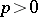. It is usually denoted by, is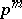-dimensional and has a basis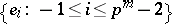with commutator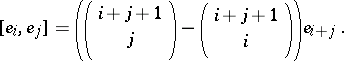It also has another basis,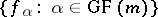(if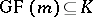), with commutator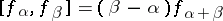, where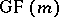is a finite field of order. Zassenhaus algebras appeared first in this form in 1939 [a8] (see also Witt algebra).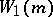is simple if(cf. also Simple algebra), has an ideal of codimensionif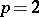,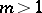, and is-dimensional non-Abelian if,. It is a Lie-algebra if and only if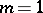. The-structure on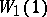can be given by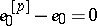,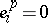,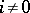. By changingto other additive subgroup of, or by changing the multiplication, one can get different algebras. For example, the multiplication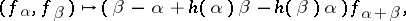whereis an additive homomorphism of finite fields, gives rise to the Albert–Zassenhaus algebra.

Suppose that all algebras and modules are finite-dimensional and that the ground fieldis an algebraically closed field of characteristic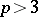.

Let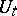be the-module defined for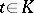on the vector space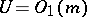by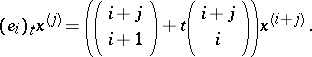For example,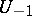andare isomorphic to the adjoint and co-adjoint modules,has an irreducible submodule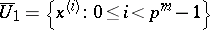, andis irreducible if. Any irreducible restricted-module is isomorphic to one of the following modules: the-dimensional trivial module; the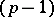-dimensional module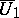; or the-dimensional module,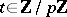,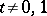. The maximal dimension of irreducible-modules is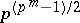, but there may be infinitely many non-isomorphic irreducible modules of given dimension. The minimal dimension of irreducible non-trivial-modules is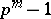, and any irreducible module of dimensionis isomorphic to. Any irreducible-module with a non-trivial action of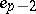is irreducible as a module over the maximal subalgebra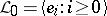. In any case, any non-restricted irreducible-module is induced by some irreducible submodule of[a3].

Any simple Lie algebra of dimensionwith a subalgebra of codimensionis isomorphic tofor some[a4]. Albert–Zassenhaus algebras have subalgebras of codimension. Any automorphism ofis induced by an admissible automorphism of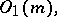i.e., by an automorphism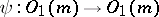such that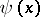is a linear combination of, where,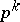,. There are infinitely many non-conjugate Cartan subalgebras (cf. also Cartan subalgebra) of dimension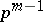if, and exactly two non-conjugate Cartan subalgebras of dimensionif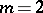[a2]. The algebra of outer derivations ofis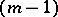-dimensional and generated by the derivations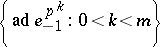. The algebrahas a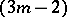-parametric deformation [a6]. A non-split central extension ofwas constructed first by R. Block in 1968 [a1]. The characteristic-infinite-dimensional analogue of this extension is well known as the Virasoro algebra. The list of irreducible-modules that have non-split extensions is the following:. All bilinear invariant forms ofare trivial, but it has a generalized Casimir element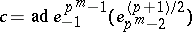. The centre of the universal enveloping algebra is generated by the-centre and the generalized Casimir elements [a7].